﻿ 水下自主机器人航向控制算法应用研究
 舰船科学技术2020, Vol. 42Issue (2): 108-114PDF

1. 中国科学院沈阳自动化研究所 机器人学国家重点实验室，辽宁 沈阳 110016;
2. 中国科学院机器人与智能制造创新研究院, 辽宁 沈阳 110016;
3. 中国科学院大学，北京 100049

Application research of heading control algorithm for AUV
LV Hou-quan1,2,3, ZHENG Rong1,2, YANG Bin1,2, LIU Bo-feng1,2, YANG Bo1,2
1. State Key Laboratory of Robotics, Shenyang Institute of Automation Chinese Academy of Science, Shenyang 110016, China;
2. Institutes for Robotics and Intelligent Manufacturing, Chinese Academy of Science, Shenyang 110016, China;
3. University of Chinese Academy of Science, Beijing 100049, China
Abstract: In order to improve the control quality of the AUV heading control system, a parameter self-adjusting PID algorithm based on fuzzy theory is studied., the algorithm adjusts the controller parameters online based on the original AUV controller so that the controller has some adaptive characteristics. The influence of fuzzy control rules for the control effect is analyzed., a scale transformation method based on nonlinear function is proposed to process the fuzzy controller input data, which simplifies the quantization process of the fuzzy controller input data. Finally, the effectiveness of the fuzzy PID control algorithm is verified by experiments on the lake., In the comparative experiment, the improved algorithm can obtain better control result in the AUV heading control. In the large angle steering, the superiority of the control algorithm is obvious: the system response is fast, overshoot is small, and the heading error is small.
Key words: AUV     heading control     PID     fuzzy control
0 引　言

1 问题描述

AUV水下运动是十分复杂的空间六自由度运动，工程应用中通常简化水平面和纵垂面运动间的耦合特性，针对两平面的运动分别设计控制器。通过泰勒级数展开法对水动力系数线性化处理，忽略质量系数矩阵中的耦合项后，可以得到平面内AUV的动力学模型如下：

 ${ A}\left[ {\begin{array}{*{20}{c}} {{{\dot v}_r}} \\ {\dot r} \\ {\dot \psi } \end{array}} \right] = {{B}}\left[ {\begin{array}{*{20}{c}} {{v_r}} \\ r \\ \psi \end{array}} \right] + \left[ {\begin{array}{*{20}{c}} {{Y_\delta }} \\ {{N_\delta }} \\ 0 \end{array}} \right]{\delta _r}\left( t \right){\text{。}}$ (1)
 ${ A} = \left[\!\! {\begin{array}{*{20}{c}} {{{m}} - {Y_{{{\dot v}_r}}}} \!\!&\!\! 0 \!\!&\!\! 0 \\ 0 \!\!&\!\! {{I_{zz}} - {N_{\dot r}}} \!\!&\!\! 0 \\ 0 \!\!&\!\! 0 \!\!&\!\! 1 \end{array}} \!\!\right],{ B} = \left[\!\! {\begin{array}{*{20}{c}} {{Y_{{v_r}}}} \!\!&\!\! {{Y_r} - m{U_0}} \!\!&\!\! 0 \\ {{N_{{v_r}}}} \!\!&\!\! {{N_r}} \!\!&\!\! 0 \\ 0 \!\!&\!\! 1 \!\!&\!\! 0 \end{array}} \!\!\right]{\text{。}}$

2 算法设计

PID控制本身的算法设计并不依赖于系统的模型，但算法的参数需要根据实际系统进行整定，是一种经典的基于反馈的线性控制器。该算法应用在非线性系统时，通常采用分段线性化的方法，在不同的工作点上分别设计控制器参数，从而实现对系统不同工作状态的控制，但工作点附近系统状态并非始终稳定，造成所获得的控制器参数难以始终保持较好的控制效果，为此有必要对传统PID算法进行改进，提高控制算法的适用性和鲁棒性。

2.1 数字PID算法

 ${{u(t)}} = {k_p} \cdot e\left( t \right) + {k_i} \cdot \mathop \int \nolimits_0^t e\left( t \right) \cdot {\rm d}t + {k_d} \cdot \frac{{{\rm d}e\left( t \right)}}{{{\rm d}t}} {\text{。}}$ (2)

 $\left\{ {\begin{array}{*{20}{c}} {{k_{p1}} = {k_p} + \Delta {k_p}} {\text{，}}\\ {{k_{i1}} = {k_i} + \Delta {k_i}} {\text{，}}\\ {{k_{d1}} = {k_d} + \Delta {k_d}} {\text{。}} \end{array}} \right.$ (3)
2.2 模糊控制算法

1）确定模糊控制器的输入变量。输出变量和论域：

2）模糊化和解模糊

 $\frac{{\mathop \sum \nolimits^ {x_i} \cdot {\mu _n}\left( x \right){\rm d}x}}{{{\mu _n}\left( x \right)}} {\text{。}}$ (4)

3）确定模糊规则和推理方法

$\vdots$

 $\begin{split} & C' = (A'{\rm and}B')^\circ \left( {\left[ {({A_1}{\rm{and}}{B_1}} \right) \to {C_1}} \right] \cup \cdot \cdot \cdot \cup \\ & \left[ {({A_n}{\rm{and}}{B_n}} \right) \to {C_n}]) = {C_1}^{\rm{'}} \cup {C_2}^{\rm{'}} \cup \cdots \cup {C_n}^{\rm{'}} \end{split}$ (5)

→”是命题联结词符号。“ $^\circ$ ”是模糊关系合成算子。

4）模糊输入量和输出量变换

2.3 模糊PID控制算法表 1 比例系数Δkp控制规则表 Tab.1 Scale factor control rules of Δkp表 2 积分系数Δki控制规则表 Tab.2 Integration coefficient control rules of Δki表 3 微分系数Δkd控制规则表 Tab.3 Differential coefficient control rules of Δkd

 ${{y(x)}} = \frac{2}{{1 + {e^{ - kx}}}} - 1 {\text{。}}$ (6)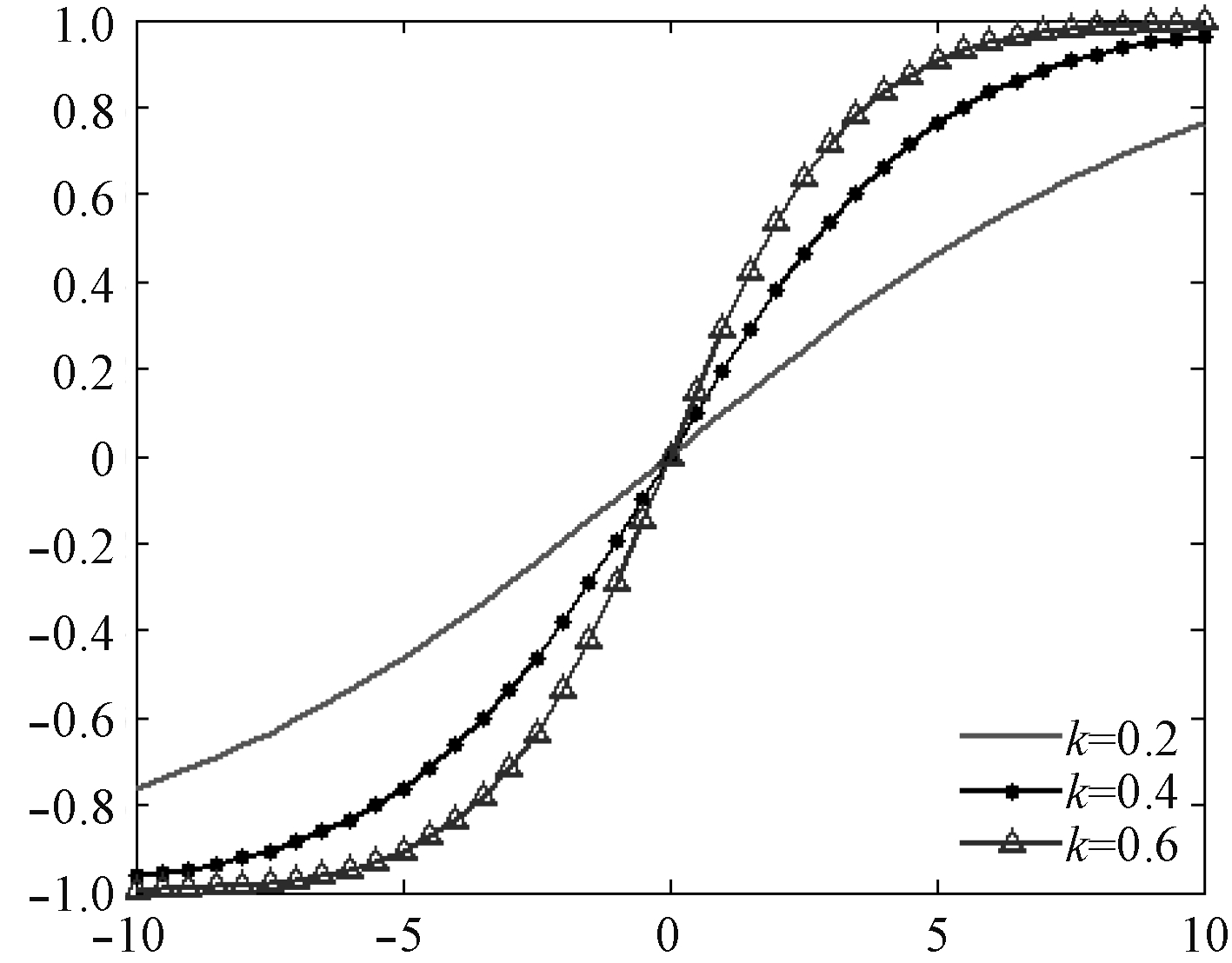图 1 尺度函数曲线 Fig. 1 Scaling function curve
3 仿真验证

3.1 Matlab仿真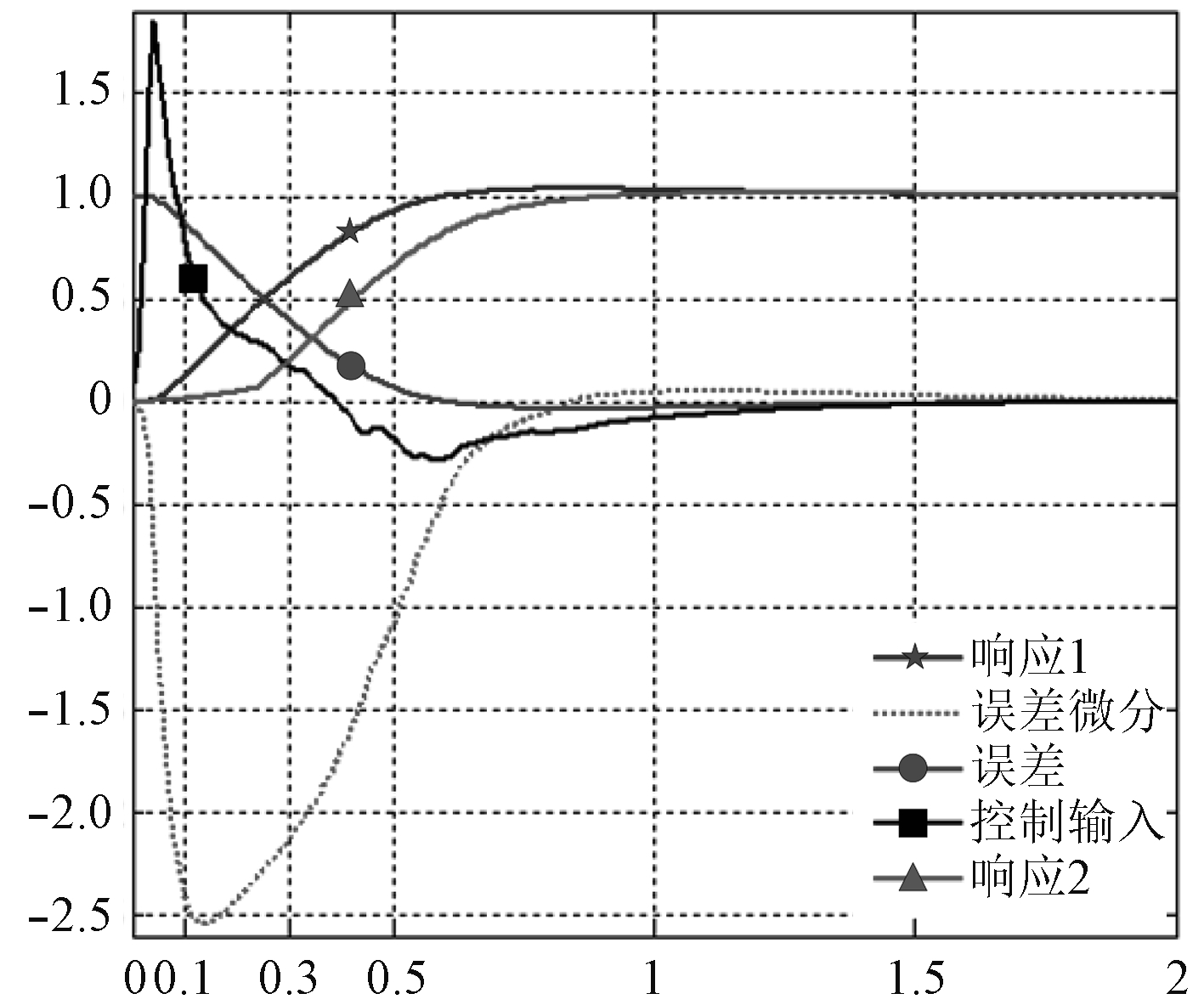图 2 系统输入与响应 Fig. 2 System input and response
 ${\rm{G}}\left( {\rm{s}} \right) = \frac{{4.718 s + 13.48}}{{0.158\;3{s^3} + 1.587{s^2} + s}} {\text{。}}$ (7)

PID控制器参数设定为：kp=1.107，ki=0，kd=0.12，得到如图2所示的系统输入和响应曲线。图中响应1曲线超调量较大，超调量3.5%，稳态时间3.21 s。为了降低系统超调并提高系统稳态时间，对响应1相对应系统控制输入、控制误差、误差的微分进行分析，各曲线如图2所示。瞬态响应阶段，为了尽快跟踪设定值，控制输入应较大，而为了减小超调，在响应没有跟上设定值时就应该降低输入，图中输入曲线满足这一规律。但注意到，在0.5～1 s过程中，误差已经趋于零时，误差的微分仍然较大，因此，在原控制规律的基础上，需要适当降低控制输入数值的大小，在比例系数规则表和微分系数规则表中对相应模糊输出进行适当的调小处理。调整后仿真得到响应2曲线。相比响应1、响应2超调量减少到1.6%，稳态时间为2.3 s，相比降低了0.91 s，但响应时间却有所增加。以上可知，在不同的使用需求下，可以通过适当调整模糊控制规则来得到不同的控制品质。

3.2 AUV数字仿真平台仿真

AUV数字仿真平台使用AUV控制程序全真模拟实际航行条件对控制算法进行仿真验证，该部分主要对公式（6）尺度变换函数中的参数k进行调整。设定航向PID参数调整量最大取值maxΔkp=20%kp，maxΔki=100%ki，maxΔkd=20%kd。AUV前向速度2节，航行深度5 m，航行规划如图3所示。AUV从A点出发，航向使命依次为：235°，225°，135°和315°，各使命航行时间均为5 min，转向角度依次增大。在相同的航行任务下，分别设定误差尺度变换函数参数ke=0.2，0.4，0.6，误差变化率尺度变换函数参数kec=0.2，0.4，0.6进行仿真测试。对不同条件下的系统动态性能进行统计，结果如表4所示。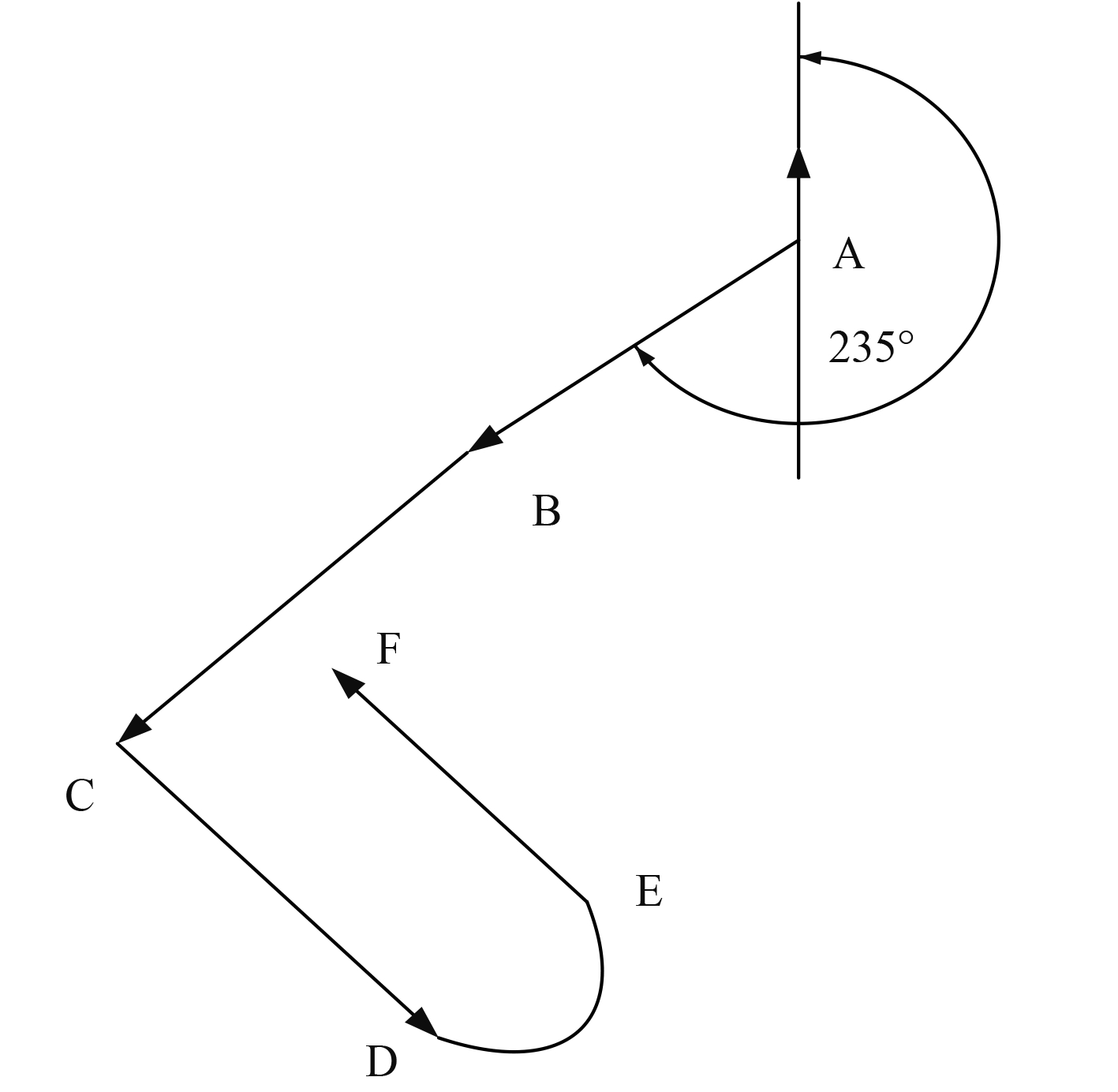图 3 航行规划图 Fig. 3 Navigation plan表 4 尺度变换函数参数取不同数值情况下的仿真结果 Tab.4 Simulation results of scale conversion function parameters taking different values表 5 改变比例项参数幅值后的仿真结果 Tab.5 Simulation result after changing the amplitude of the proportional term parameter

4 航行试验

2018年12月在浙江省千岛湖进行了湖上试验，试验用载体为鱼雷外形重型AUV，长度8.4 m左右，直径534 mm，最大下潜深度200 m，空气中重量1.6 t。该AUV为十字形尾舵，并配有垂直槽道推进器用于低速时的深度控制。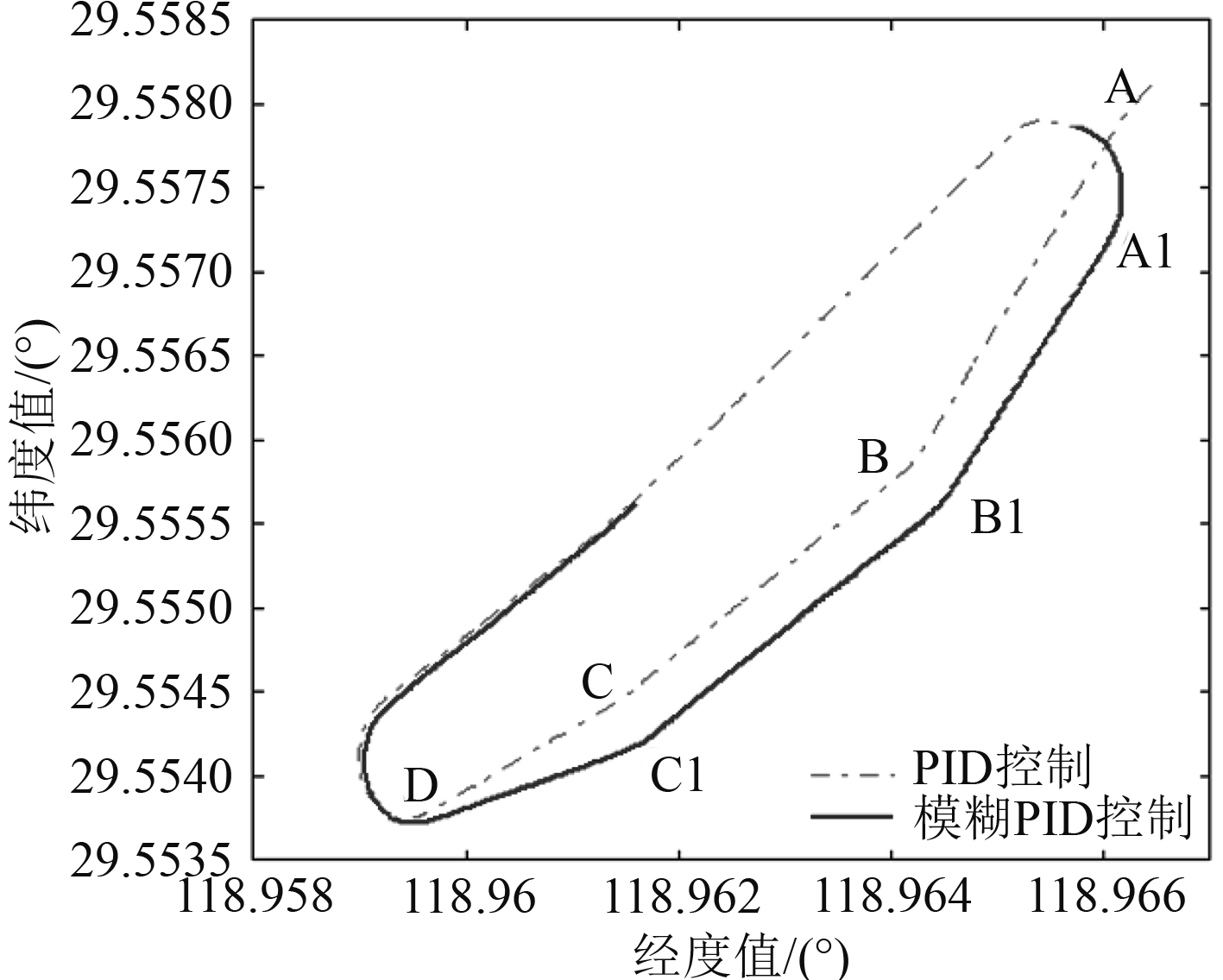图 4 AUV 航迹图 Fig. 4 AUV Navigation path recorder chart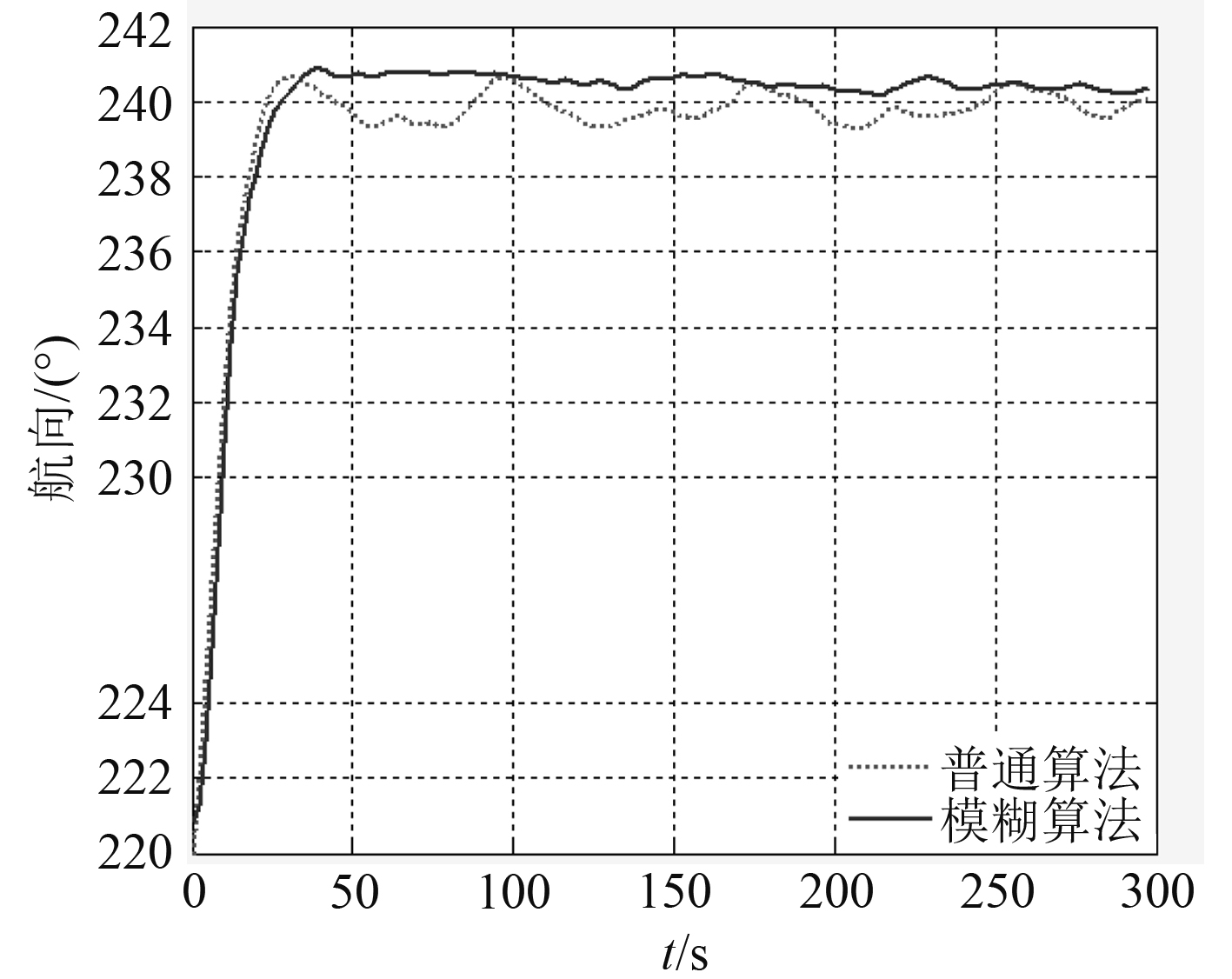图 5 小角度转向AUV航向变化曲线 Fig. 5 Small angle steering AUV course change curve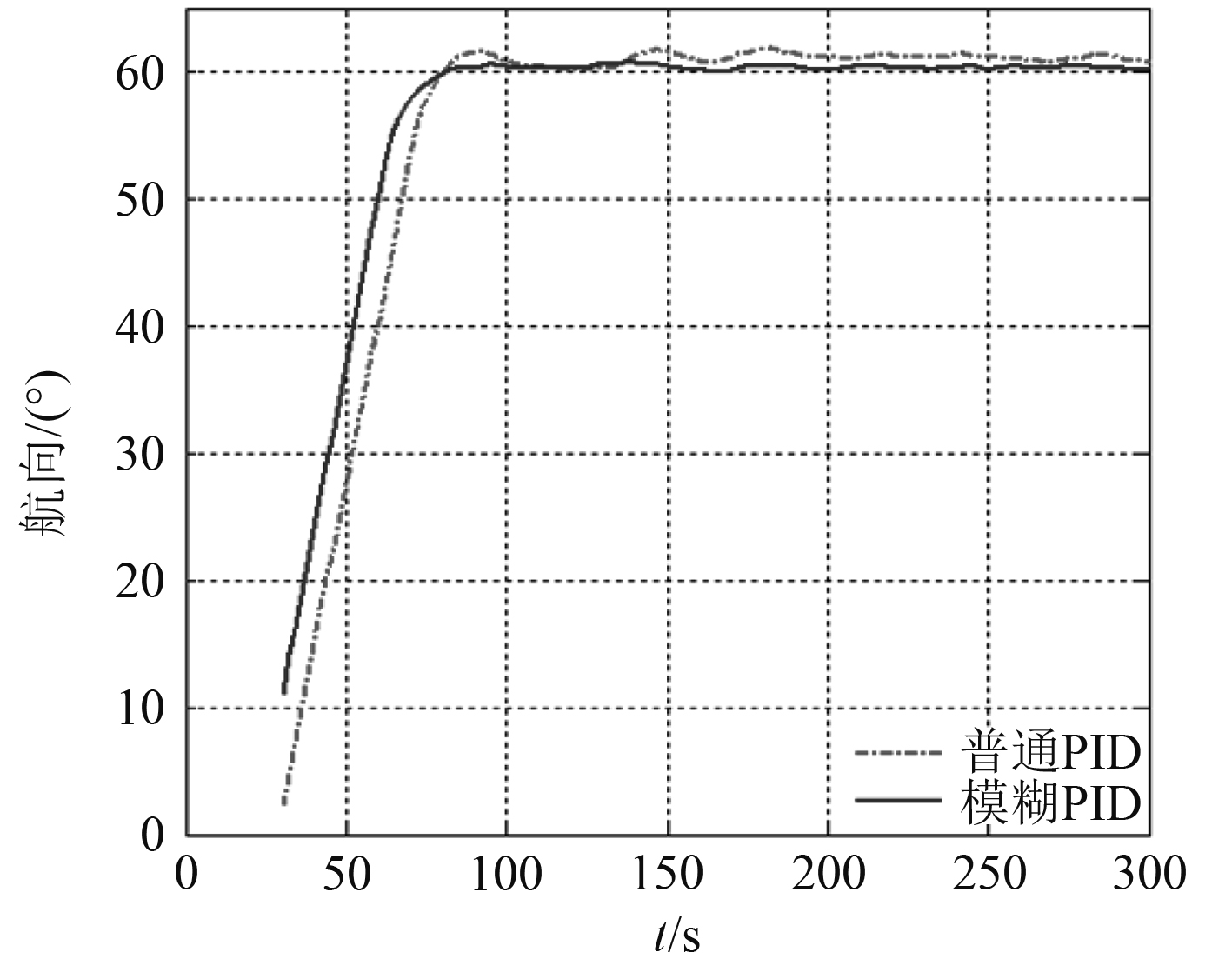图 6 大角度转向AUV航向变化曲线 Fig. 6 Large angle steering AUV course change curve表 6 大角度转向时系统响应数据对比 Tab.6 Comparison of system response during large angle steering

5 结　语

  Y. SATO, Ma ki, Toshihiro, Ku me, et al. Path replanning method foran AUV in natural hydrothermal vent fields: toward 3D imaging of a hydrothermal chimney[J]. Marine Technology Society, 2014, 48(3): 104-114. DOI:10.4031/MTSJ.48.3.5  CHOYEKH M K, NaOMI S, et al. Vertical water column survey in the gulf of mexico using autonomous underwater vehicle SOTAB-I[J]. Marine Technology Society Journal, 2015, 49(3): 88-101. DOI:10.4031/MTSJ.49.3.8  严浙平, 周佳加. 水下无人航行器控制技术[M]. 北京: 国防工业出版社, 2015: 153-154.  贾鹤鸣. 基于反步法的欠驱动UUV空间目标跟踪非线性控制方法研究[D]. 哈尔滨: 哈尔滨工程大学. 2012. JIA H M. Study of spatial tracking nonlinear control of underactuated UUV based on backstepping[D]. Harbin: Harbin Engineering University. 2012.  LEKKAS A, FOSSEN T I. A Quaternion-based LOS guidance scheme for path following of AUVs[J]. Ifac Proceedings Volumes, 2013, 46(33): 245-250. DOI:10.3182/20130918-4-JP-3022.00070  AGUIAR A P, PASCOAL A M. Dynamic positioning and way-point tracking of underactuated AUVs in the presence of ocean currents[C]// IEEE Conference on Decision & Control. IEEE, 2002.  YANG R, CLEMENT B, et al. Robust heading control and its application to ciscrea underwater vehicle[C]// Oceans. IEEE, 2015.  MASHHAD A M, MASHHADI S K M. H infinity robust controller comparison with PD like fuzzy logic controller for an AUV control[C]// Fuzzy & Intelligent Systems. IEEE, 2016.  姜春萌. 自主水下机器人智能控制与参数优化技术研究[D]. 哈尔滨: 哈尔滨工程大学. 2018. JIANG C M. Research on AUV Intelligent Control and Parameter Optimization Technology[D]. Harbin Engineering University. 2018.  HEALEY A J. Multivariable sliding mode control for autonomous diving and steering of unmanned underwater vehicles[J]. IEEE J. Oceanic Eng, 1993, 18(3): 327-339. DOI:10.1109/JOE.1993.236372  MARCO D B, HEALEY A J. Command, control, and navigation experimental results with the NPS ARIES AUV[J]. IEEE Journal of Oceanic Engineering, 2001, 26(4): 466-476. DOI:10.1109/48.972079  孙增圻, 邓志东, 张再兴. 智能控制理论与技术[M]. 北京: 清华大学出版社, 2011: 17.  从爽. 智能控制系统及其应用[M]. 合肥: 中国科学技术大学出版社, 2013: 138-143.  徐德民, 严卫生. 鱼雷控制系统计算机辅助分析设计与仿真[M]. 西安: 西北工业大学出版社, 2000: 79-80.